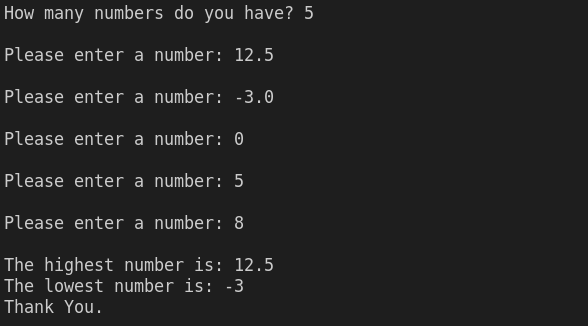Write a C++ program that receives the total number of integers (N) from the user. Then, the program will ask the user for N real numbers. By applying for a loop, your program should find and display the largest and the lowest of the numbers. Give a proper message for invalid user input, as shown below:

#Sample Program Run#1

How many numbers do you have? > -3

Sorry, you have entered an invalid input.

Thank You.

#Sample Program Run#2

How many numbers do you have? > 0

Oops, you don't have any number for me to process.

Thank You.

#Sample Program Run#3

How many numbers do you have? > 5

Please enter a number_1 --> 12.5

Please enter a number_2 --> -3.2

Please enter a number_3 --> 0

Please enter a number_4 --> 5

Please enter a number_5 --> 8.5

The lowest number is -3.20

The highest number is 12.50

Thank You

#include <iostream>
using namespace std;

int main()
{
int choice;
float lowest = 0.0;
float highest = 0.0;
float number;
cout<<"How many numbers do you have? ";
cin>>choice;
if (choice < 0)
{
cout<<"Sorry, you have entered an invalid input.\n"
<<"Thank You.\n";
}
else if (choice == 0)
{
cout<<"Opps, you don't have any number for me to process.\n";
cout<<"Thank You.\n";
}
else
{
for (int i = 1; i <= choice; i++)
{
cin>>number;
if(i ==1)
{
highest = number;
lowest = number;
}
if (highest < number)
{
highest = number;
}
else if (lowest > number)
{
lowest = number;
}
}
cout<<"\nThe highest number is: "<<highest;
cout<<"\nThe lowest number is: "<<lowest;
cout<<"\nThank You.";
}
return 0;
}cpp programming exercise with solution